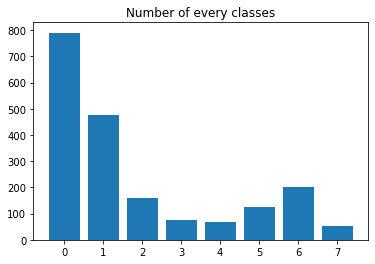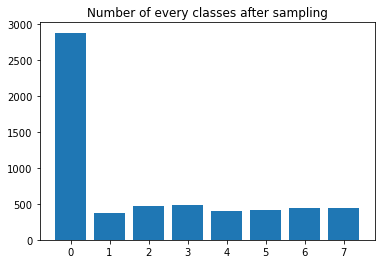# Weighted cross entropy

Hello everyone, I am doing a deep learning project which has imbalanced class dataset.
So, I am trying to use weighted cross entropy with soft dice loss.
However, I have a question regarding use of weighted ce.
I usually set my weights for classes as 1/no.instance which seems to be correct I think.
This should work well as it counts every instances for each class but, this seems to be not working so well compare to when I approximately set the weights for each class.
What could be a reason for my model performing worse when I weight classes by 1/number of occurrences in each image compare to giving weights by my random prediction?
Thank you and I look forward to hearing from someone for the help!

1 Like

I think setting the initial weights as `1/class_count` is a viable initial value, but not necessarily the most suitable for your use case and I think it’s the right approach to play around with these values to find a “sweet spot” which fits your use case well.

2 Likes

aha i see.
@ptrblck, just one more question about the loss function.
For the case of multi class focal loss, is alpha which is the hyperparameter just the same weight as the weighted cross entropy?
Thank you!

I’m not sure what `alpha` refers to, but the focal loss would be weigthed by `(1 - pred)**gamma`, if I remember it correctly. In this case the weighting isn’t a static value, but depends on the output probability of the model for the current target, such that “well-classified samples” get a lower loss than the wrongly classified ones.

Hi, thank you so much for the help.
I just read the paper again and i think alpha is a list of weight for classes.
Currently, my dataset is hugely imbalanced and when I sometimes train my model, validation accuracy and iou stay exactly same for every epoch. Do you know what might have caused this and how to solve it?
Could it be due to overfitting?

Is the training accuracy and IOU still decreasing while the validation metrics stay the same?
In that case, yes it sounds like overfitting.

The problem only occurs when I set learning rate scheduler.
Could lr_scheduler cause sucb the problem?
Also, Is the weight_decay parameter equivalent to L2 regularization?

Actually training accuracy and IOU don’t decrease when this problem happens which is really weird.

Your learning rate scheduler might reduce the learning rate too far and maybe the training just gets stuck?
Did you try to remove the scheduler and does the training benefit from it?

`weight_decay` will add L2 regularization to all parameters for standard SGD. Note that for certain optimizers (such as `Adam`) the weight decay is not equal to L2 regularization as explained in Decoupled Weight Decay Regularization, which is why `AdamW` was implemented.

@ptrblck, thank you so much for the help!!
I have been using the code below for optimizer:

``````optimizer = AdamW(model.parameters(), 0.01,weight_decay=1e-4)
lmbda = lambda epoch: 0.95
scheduler = torch.optim.lr_scheduler.MultiplicativeLR(optimizer, lr_lambda=lmbda)
``````

I presume this lr_scheduler works by lr*0.95 for every epoch right?
It works fine without scheduler, but when I add the scheduler, it starts to overfitting for some reason and it happens from epoch 1 so I am not sure whether this is a overfitting problem.
Also, this problem is solved by adding weight_decay parameter in AdamW but I am not sure why.

Furthermore, I am currently facing a huge class imbalance problem in my semantic segmentation task.The original data looks as above where 0-7 are classes.
To solve this, other than using loss functions, I have also tried doing oversampling.
This looks as below and unfortunately I can not make class 0 in same range as others as class 0 appears in every dataset.Do you think the above could help to solve the imbalance problem?

Yes, that should be the case. You can double check it by printing the learning rates:

``````[...]
optimizer.step()
scheduler.step()
print(optimizer.param_groups['lr'])
print(scheduler.get_last_lr())
``````

You could certainly try your oversampling approach, but currently you are creating a lot of class0 samples, so your model might overfit to this class even more now.

@ptrblck, thank you for the reply.
I know, the class 0 has increased even more now, but the problem is that the class 0 appears in every single image as it refers to background, so it is inevitable I think if I use oversampling(with a few augmentation methods) technique.
I am currently training my model, but seems that the training iou has significantly increased but not so much with the validation iou yet.
Do you think I shouldn’t use the oversampling with augmentation technique in my application and rather leave my original dataset as it was?